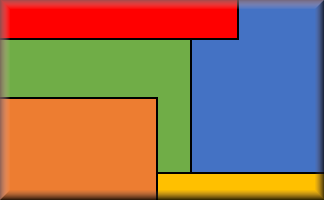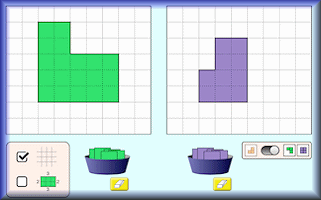#Find the length and width of a rectangle if its perimeter is 30cm and area is 50cm2.

What are the dimensions of a rectangle whose perimeter is 74cm and area is 312cm2.

Find the dimensions of a rectangle whose perimeter is 33cm and area is 65cm2.

Find the dimensions of all the rectangles whose perimeters in cm are twice the value of their areas in cm2.

## A Mathematics Lesson Starter Of The Day

• Transum,
•
• The first three problems are in increasing order of difficulty. The first can probably be guessed, the second needs more of a strategy as the numbers are larger and the third involves a fraction so probably requires the application of a more generalised method. Refreshing the page generates three different rectangles to further consolidate any techniques learned in the first round. The fourth problem is only for Nobel prize candidates!
• Simon Fletcher, Bolton UK
•
• 52 cm rectangle perimeter 105 area. Is this correct? I've checked and can't find a solution.
• Transum,
•
• Hello Simon, so you are looking for two numbers (the height and the width) that add up to half the perimeter, 26cm, and multiply together to give an area of 105cm2.
We can write two simultaneous equations showing this information:
h + w = 26
hw = 105
We can combine the information in these two equations and eliminate one of the unknowns:
From the first equation we see that h = 26 – w
Substituting this into the second equation gives (26 – w)w = 105
Expanding the brackets gives 26w - w2=105
Rearranging gives w2 - 26w + 105 = 0
This is a quadratic equation which can be solved by factorising
(w – 5)(w – 21) = 0
So w could be 5cm in which case h would be 21cm or, w could be 21cm in which case h would be 5cm.
• Audrey Macmillan, @audproctor77
•
• Clarifying area/perimeter confusion using a Transum Starter. Great #mathschat on squares and rows.

How did you use this starter? Can you suggest how teachers could present or develop this resource? Do you have any comments? It is always useful to receive feedback and helps make this free resource even more useful for Maths teachers anywhere in the world.

Previous Day | This starter is for 3 April | Next Day

3 and 3/2

4 and 4/3

5 and 5/4

6 and 6/5

7 and 7/6

8 and 8/7

9 and 9/8

10 and 10/9

Note to teacher: Doing this activity once with a class helps students develop strategies. It is only when they do this activity a second time that they will have the opportunity to practise those strategies. That is when the learning is consolidated. Click the button above to regenerate another version of this starter from random numbers.

Your access to the majority of the Transum resources continues to be free but you can help support the continued growth of the website by doing your Amazon shopping using the links on this page. Below is an Amazon link. As an Amazon Associate I earn a small amount from qualifying purchases which helps pay for the upkeep of this website.

Educational Technology on AmazonTeacher, do your students have access to computers such as tablets, iPads or Laptops?  This page was really designed for projection on a whiteboard but if you really want the students to have access to it here is a concise URL for a version of this page without the comments: Transum.org/go/?Start=April3 However it would be better to assign one of the student interactive activities below.Here are URLs which will take them to related student activities.

Transum.org/go/?to=OblongsTransum.org/go/?to=AreaBuilderFor All: